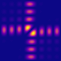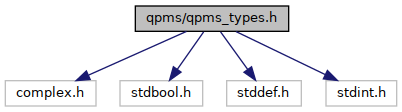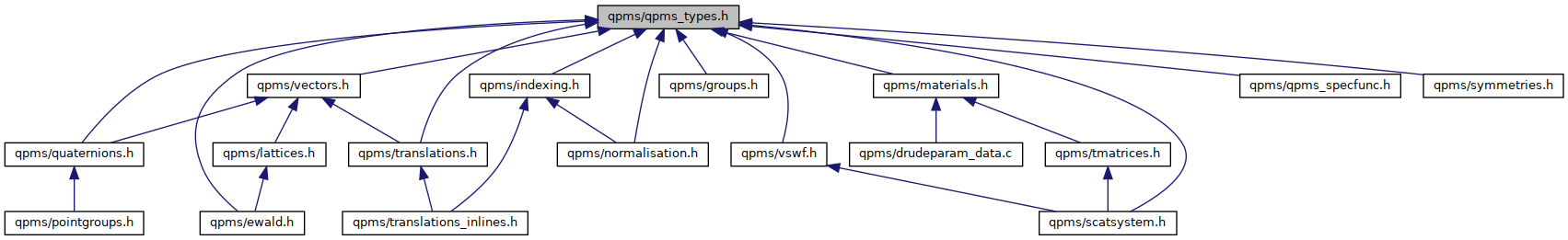QPMS Electromagnetic multiple scattering library and toolkit.
qpms_types.h File Reference

Common qpms types. More...

#include <complex.h>
#include <stdbool.h>
#include <stddef.h>
#include <stdint.h>
Include dependency graph for qpms_types.h:This graph shows which files directly or indirectly include this file:Go to the source code of this file.

## Data Structures

struct  cart3_t

struct  ccart3_t

struct  ccart3_pair
3D complex vector pair (represents the E, H fields). More...

struct  cart2_t

struct  sph_t

struct  csph_t

struct  csphvec_t
3D complex vector components in local spherical basis. See also vectors.h. More...

struct  pol_t

union  anycoord_point_t
Union type capable to contain various 1D, 2D and 3D coordinates. More...

struct  qpms_quat_t
Quaternion type. More...

struct  qpms_quat4d_t
Quaternion type as four doubles. More...

struct  qpms_irot3_t
3D improper rotations represented as a quaternion and a sign of the determinant. More...

struct  qpms_vswf_set_spec_t
Specifies a finite set of VSWFs. More...

struct  qpms_tmatrix_t
A T-matrix. More...

struct  qpms_pointgroup_t
Full characterisation of a 3D point group. More...

struct  qpms_abstract_tmatrix_t
An abstract T-matrix without actual elements, but with info about particle symmetry. More...

struct  qpms_epsmu_t
A type holding electric permittivity and magnetic permeability of a material. More...

## Macros

#define M_PI_2   (1.570796326794896619231321691639751442098584699687552910487L)

#define M_PI   (3.14159265358979323846264338327950288419716939937510582097494L)

#define M_SQRT2   (1.41421356237309504880168872420969807856967187537694807317668L)

#define M_SQRTPI   (1.77245385090551602729816748334114518279754945612238712821381L)

#define QPMS_NO_IRREP   ((qpms_iri_t) -1)
Constant labeling that no irrep decomposition is done (full system solved instead).

#define lmcheck(l, m)   assert((l) >= 1 && abs(m) <= (l))

## Typedefs

typedef int qpms_lm_t

typedef int qpms_l_t
Type for spherical harmonic degree l.

typedef qpms_lm_t qpms_m_t
Type for spherical harmonic order m.

typedef size_t qpms_y_t
Type for the (l, m) multiindex of transversal (M or N -type) VSWFs. More...

typedef size_t qpms_y_sc_t
Type for the (l, m) multiindex of spherical harmonics, including (0, 0). More...

typedef unsigned long long qpms_uvswfi_t
Exhaustive index type for VSWF basis functions. More...

typedef struct cart3_t cart3_t

typedef struct ccart3_t ccart3_t

typedef struct ccart3_pair ccart3_pair
3D complex vector pair (represents the E, H fields).

typedef struct cart2_t cart2_t

typedef struct sph_t sph_t

typedef struct csph_t csph_t

typedef struct csphvec_t csphvec_t

typedef struct pol_t pol_t

typedef union anycoord_point_t anycoord_point_t
Union type capable to contain various 1D, 2D and 3D coordinates. More...

typedef struct qpms_quat_t qpms_quat_t
Quaternion type. More...

typedef struct qpms_quat4d_t qpms_quat4d_t
Quaternion type as four doubles. More...

typedef struct qpms_irot3_t qpms_irot3_t
3D improper rotations represented as a quaternion and a sign of the determinant. More...

typedef struct qpms_vswf_set_spec_t qpms_vswf_set_spec_t
Specifies a finite set of VSWFs. More...

typedef int32_t qpms_ss_tmi_t
T-matrix index used in qpms_scatsys_t and related structures.

typedef int32_t qpms_ss_tmgi_t
T-matrix generator index used in qpms_scatsys_t and related structures.

typedef int32_t qpms_ss_pi_t
Particle index used in qpms_scatsys_t and related structures.

typedef int qpms_gmi_t

typedef int qpms_iri_t

typedef const char * qpms_permutation_t
Permutation representation of a group element. More...

typedef struct qpms_tmatrix_t qpms_tmatrix_t
A T-matrix. More...

typedef struct qpms_pointgroup_t qpms_pointgroup_t
Full characterisation of a 3D point group.

typedef struct qpms_abstract_tmatrix_t qpms_abstract_tmatrix_t
An abstract T-matrix without actual elements, but with info about particle symmetry.

typedef struct qpms_epsmu_t qpms_epsmu_t
A type holding electric permittivity and magnetic permeability of a material.

## Enumerations

enum  qpms_vswf_type_t { QPMS_VSWF_ELECTRIC = 2 , QPMS_VSWF_MAGNETIC = 1 , QPMS_VSWF_LONGITUDINAL = 0 }
Codes of the VSWF types (electric/N, magnetic/M, longitudinal/L). More...

enum  qpms_errno_t {
QPMS_SUCCESS = 0 , QPMS_ERROR = -1 , QPMS_FAILURE = QPMS_ERROR , QPMS_ENOMEM = 8 ,
QPMS_ESING = 21 , QPMS_NAN_ENCOUNTERED = 1024
}
Error codes / return values for certain numerical functions. More...

enum  qpms_normalisation_t {
QPMS_NORMALISATION_UNDEF = 0 , QPMS_NORMALISATION_INVERSE = 1 , QPMS_NORMALISATION_REVERSE_AZIMUTHAL_PHASE = 2 , QPMS_NORMALISATION_SPHARM_REAL = 4 ,
QPMS_NORMALISATION_CSPHASE = 8 , QPMS_NORMALISATION_M_I = 16 , QPMS_NORMALISATION_M_MINUS = 32 , QPMS_NORMALISATION_N_I = 64 ,
QPMS_NORMALISATION_N_MINUS = 128 , QPMS_NORMALISATION_L_I = 256 , QPMS_NORMALISATION_L_MINUS = 512 , QPMS_NORMALISATION_NORM_BITSTART = 65536 ,
QPMS_NORMALISATION_NORM_POWER = QPMS_NORMALISATION_NORM_BITSTART * 1 , QPMS_NORMALISATION_NORM_SPHARM = QPMS_NORMALISATION_NORM_BITSTART * 3 , QPMS_NORMALISATION_NORM_NONE = QPMS_NORMALISATION_NORM_BITSTART * 2 , QPMS_NORMALISATION_NORM_BITS ,
QPMS_NORMALISATION_CONVENTION_KRISTENSSON_REAL , QPMS_NORMALISATION_CONVENTION_KRISTENSSON , QPMS_NORMALISATION_CONVENTION_SCUFF , QPMS_NORMALISATION_DEFAULT = QPMS_NORMALISATION_CONVENTION_KRISTENSSON
}
Vector spherical wavefuction normalisation and phase convention codes. More...

enum  qpms_bessel_t {
QPMS_BESSEL_REGULAR = 1 , QPMS_BESSEL_SINGULAR = 2 , QPMS_HANKEL_PLUS = 3 , QPMS_HANKEL_MINUS = 4 ,
QPMS_BESSEL_UNDEF = 0
}
Bessel function kinds. More...

enum  qpms_coord_system_t {
QPMS_COORDS_CART1 = 64 , QPMS_COORDS_POL = 128 , QPMS_COORDS_SPH = 256 , QPMS_COORDS_CART2 = 512 ,
QPMS_COORDS_CART3 = 1024 , QPMS_COORDS_BITRANGE
}
Enum codes for common coordinate systems. More...

enum  qpms_pointgroup_class {
QPMS_PGS_CN , QPMS_PGS_S2N , QPMS_PGS_CNH , QPMS_PGS_CNV ,
QPMS_PGS_DN , QPMS_PGS_DND , QPMS_PGS_DNH , QPMS_PGS_T ,
QPMS_PGS_TD , QPMS_PGS_TH , QPMS_PGS_O , QPMS_PGS_OH ,
QPMS_PGS_I , QPMS_PGS_IH , QPMS_PGS_CINF , QPMS_PGS_CINFH ,
QPMS_PGS_CINFV , QPMS_PGS_DINF , QPMS_PGS_DINFH , QPMS_PGS_SO3 ,
QPMS_PGS_O3
}
Classification of a 3D point group. More...

## Functions

static int qpms_normalisation_t_csphase (qpms_normalisation_t norm)
Determine whether the convention includes Condon-Shortley phase (-1) or not (+1).

## Detailed Description

Common qpms types.

## ◆ anycoord_point_t

 typedef union anycoord_point_t anycoord_point_t

Union type capable to contain various 1D, 2D and 3D coordinates.

Usually combined with qpms_coord_system_t.

## ◆ cart2_t

 typedef struct cart2_t cart2_t

## ◆ qpms_irot3_t

 typedef struct qpms_irot3_t qpms_irot3_t

3D improper rotations represented as a quaternion and a sign of the determinant.

See quaternions.h for "methods".

## ◆ qpms_permutation_t

 typedef const char* qpms_permutation_t

Permutation representation of a group element.

For now, it's just a string of the form "(0,1)(3,4,5)"

## ◆ qpms_quat4d_t

 typedef struct qpms_quat4d_t qpms_quat4d_t

Quaternion type as four doubles.

See quaternions.h for "methods".

## ◆ qpms_quat_t

 typedef struct qpms_quat_t qpms_quat_t

Quaternion type.

Internaly represented as a pair of complex numbers, $$Q_a = Q_1 + iQ_z, Q_b = Q_y + i Q_x$$.

See quaternions.h for "methods".

## ◆ qpms_tmatrix_t

 typedef struct qpms_tmatrix_t qpms_tmatrix_t

A T-matrix.

In the future, I might rather use a more abstract approach in which T-matrix is a mapping (function) of the field expansion coefficients. So the interface might change. For now, let me stick to the square dense matrix representation.

## ◆ qpms_uvswfi_t

 typedef unsigned long long qpms_uvswfi_t

Exhaustive index type for VSWF basis functions.

Carries information about the wave being of M/N/L (magnetic, electric, or longitudinal) type, as well as the wave's degree and order (l, m).

The formula is 4 * (qpms_y_sc_t) y_sc + (qmps_vswf_type_t) type_code, but don't rely on this and use the functions qpms_tmn2uvswfi() and qpms_uvswfi2tmn() from qpms_types.h instead as the formula might change in future versions.

## ◆ qpms_vswf_set_spec_t

 typedef struct qpms_vswf_set_spec_t qpms_vswf_set_spec_t

Specifies a finite set of VSWFs.

When for example not all the M and N -type waves up to a degree lMax need to be computed, this will specify the subset.

A typical use case would be when only even/odd fields wrt. z-plane mirror symmetry are considered.

See vswf.h for "methods".

## ◆ qpms_y_sc_t

 typedef size_t qpms_y_sc_t

Type for the (l, m) multiindex of spherical harmonics, including (0, 0).

This differs from qpms_y_t by being shifted by one and including the l = 0 option. Suitable also for scalar and longitudinal waves. Corresponds to the l -primary, m -secondary ordering, i.e.

$y = 0: l = 0, m = 0,$

$y = 1: l = 1, m = -1,$

$y = 2: l = 1, m = 0,$

$y = 3: l = 1, m = +1,$

$y = 4: l = 2, m = -2,$

...

## ◆ qpms_y_t

 typedef size_t qpms_y_t

Type for the (l, m) multiindex of transversal (M or N -type) VSWFs.

This corresponds to the typical memory layout for various coefficient etc. Corresponds to the l-primary, m-secondary ordering, i.e.

$y = 0: l = 1, m = -1,$

$y = 1: l = 1, m = 0,$

$y = 2: l = 1, m = +1,$

$y = 3: l = 2, m = -2,$

...

## ◆ qpms_bessel_t

 enum qpms_bessel_t

Bessel function kinds.

Enumerator
QPMS_BESSEL_REGULAR

regular (spherical) Bessel function j (Bessel function of the first kind)

QPMS_BESSEL_SINGULAR

singular (spherical) Bessel function y (Bessel function of the second kind)

QPMS_HANKEL_PLUS

(spherical) Hankel function $$h_1 = j + iy$$

QPMS_HANKEL_MINUS

(spherical) Hankel function $$h_2 = j - iy$$

QPMS_BESSEL_UNDEF

invalid / unspecified kind

## ◆ qpms_coord_system_t

 enum qpms_coord_system_t

Enum codes for common coordinate systems.

Enumerator
QPMS_COORDS_CART1

1D cartesian (= double).

QPMS_COORDS_POL

2D polar.

QPMS_COORDS_SPH

3D spherical.

QPMS_COORDS_CART2

2D cartesian.

QPMS_COORDS_CART3

3D cartesian.

QPMS_COORDS_BITRANGE

Convenience bitmask (not a valid flag!).

## ◆ qpms_errno_t

 enum qpms_errno_t

Error codes / return values for certain numerical functions.

Those with values between -2 and 32 are subset of the GSL error codes.

Enumerator
QPMS_SUCCESS

Success.

QPMS_ERROR

Unspecified error.

QPMS_ENOMEM

Out of memory.

QPMS_ESING

Apparent singularity detected.

QPMS_NAN_ENCOUNTERED

NaN value encountered in data processing.

## ◆ qpms_normalisation_t

 enum qpms_normalisation_t

Vector spherical wavefuction normalisation and phase convention codes.

Throughout the literature, various conventions for VSWF bases are used. These bit flags are used by the functions declared in normalisation.h that return the appropriate convention-dependent factors.

See VSWF conventions for comparison of the various conventions used.

Enumerator
QPMS_NORMALISATION_UNDEF

Convention undefined. This should not happen.

QPMS_NORMALISATION_INVERSE

Flag indicating that qpms_normalisition_factor_* should actually return values inverse to the default.

QPMS_NORMALISATION_REVERSE_AZIMUTHAL_PHASE

Flag indicating inversion of the asimuthal phase for complex spherical harmonics (i.e. $$e^{-im\phi}$$ instead of $$e^{im\phi}$$.

QPMS_NORMALISATION_SPHARM_REAL

Flag indicating use of the real spherical harmonics.

If QPMS_NORMALISATION_REVERSE_AZIMUTHAL_PHASE is unset, negative m correspond to sine in the asimuthal factor; if set, undefined behaviour.

QPMS_NORMALISATION_CSPHASE

Flag indicating usage of Condon-Shortley phase.

If set, the Ferrers functions and everything derived from them (spherical harmonics, VSWFs) will include a $$(-1)^m$$ factor.

On implementation level, this means that the relevant gsl_sf_legendre_*_e() functions will be called with argument csphase = -1. instead of +1..

QPMS_NORMALISATION_M_I

Include an additional i -factor into the magnetic waves.

QPMS_NORMALISATION_M_MINUS

Include an additional $$-1$$ -factor into the magnetic waves.

QPMS_NORMALISATION_N_I

Include an additional i -factor into the electric waves.

QPMS_NORMALISATION_N_MINUS

Include an additional $$-1$$ -factor into the magnetic waves.

QPMS_NORMALISATION_L_I

Include an additional i -factor into the longitudinal waves.

QPMS_NORMALISATION_L_MINUS

Include an additional $$-1$$ -factor into the longitudinal waves.

QPMS_NORMALISATION_NORM_POWER

The VSWFs shall be power-normalised. This is the "default".

Power normalisation is used e.g. in  (complex spherical harmonics with Condon-Shortley phase) or  (real spherical harmonics). This is also the reference for all the other normalisation conventions, meaning that qpms_normalisation_factor_M() and qpms_normalisation_factor_N() shall always return 1. + 0.*I if norm == QPMS_NORMALISATION_NORM_POWER.

QPMS_NORMALISATION_NORM_SPHARM

The VSWFs shall be normalised as in  .

This includes a $$\sqrt{l(l+1)}$$ factor compared to the power normalisation.

QPMS_NORMALISATION_NORM_NONE

The VSWFs shall be created using spherical harmonics without any normalisation. Do not use.

This includes a

$\sqrt{l(l+1)} \left(\frac{(2l+1)}{4\pi}\frac{(l-m)!}{(l+m)!}\right)^{-\frac{1}{2}}$

factor compared to the power normalisation.

Note that this has no sense whatsoever for real spherical harmonics. Again, do not use this.

QPMS_NORMALISATION_CONVENTION_KRISTENSSON_REAL

VSWF convention used in .

QPMS_NORMALISATION_CONVENTION_KRISTENSSON

VSWF convention used in .

QPMS_NORMALISATION_CONVENTION_SCUFF

VSWF convention used in SCUFF-EM .

QPMS_NORMALISATION_DEFAULT

Default VSWF convention. We might encourage the compiler to expect this one.

## ◆ qpms_pointgroup_class

Classification of a 3D point group.

Enumerator
QPMS_PGS_CN

Rotational symmetry $$\mathrm{C_{n}}$$.

QPMS_PGS_S2N

Rotoreflectional symmetry $$\mathrm{S_{2n}}$$.

QPMS_PGS_CNH

Rotational symmetry with horizontal reflection $$\mathrm{C_{nh}}$$.

QPMS_PGS_CNV

Pyramidal symmetry $$\mathrm{C_{nv}}$$.

QPMS_PGS_DN

Dihedral symmetry $$\mathrm{D_{n}}$$.

QPMS_PGS_DND

Antiprismatic symmetry $$\mathrm{D_{nd}}$$.

QPMS_PGS_DNH

Prismatic symmetry $$\mathrm{D_{nh}}$$.

QPMS_PGS_T

Chiral tetrahedral symmetry $$\mathrm{T}$$.

QPMS_PGS_TD

Full tetrahedral symmetry $$\mathrm{T_d}$$.

QPMS_PGS_TH

Pyritohedral symmetry $$\mathrm{T_h}$$.

QPMS_PGS_O

Chiral octahedral symmetry $$\mathrm{O_h}$$.

QPMS_PGS_OH

Full octahedral symmetry $$\mathrm{O_h}$$.

QPMS_PGS_I

Chiral icosahedral symmetry $$\mathrm{I}$$.

QPMS_PGS_IH

Full icosahedral symmetry $$\mathrm{I_h}$$.

QPMS_PGS_CINF

$$\mathrm{C_\infty}$$

QPMS_PGS_CINFH

$$\mathrm{C_{\infty h}}$$

QPMS_PGS_CINFV

$$\mathrm{C_{\infty v}}$$

QPMS_PGS_DINF

$$\mathrm{D_\infty}$$

QPMS_PGS_DINFH

$$\mathrm{D_{\infty h}}$$

QPMS_PGS_SO3

Special orthogonal group.

QPMS_PGS_O3

Orthogonal group.

## ◆ qpms_vswf_type_t

 enum qpms_vswf_type_t

Codes of the VSWF types (electric/N, magnetic/M, longitudinal/L).

Enumerator
QPMS_VSWF_ELECTRIC

"Electric" (N -type) transversal wave.

QPMS_VSWF_MAGNETIC

"Magnetic" (M -type) transversal wave.

QPMS_VSWF_LONGITUDINAL

Longitudinal (L -type) wave (not relevant for radiation).